Next: Semi-infinite body heated at Up: EXAMPLES, TEMPERATURE FROM GF Previous: EXAMPLES, TEMPERATURE FROM GF

## 1D infinite body with initial condition.

Consider the temperature caused by a spatially-varying initial condition in an infinite 1D body. The temperature satisfies the following equations: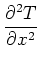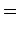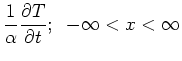(1)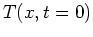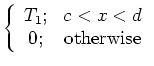This case has number X00T5. The solution for the temperature is given by the initial condition term of the GF solution equation: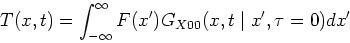(2)

Because the initial condition is zero over most of the domain the integral may be carried out over the non-zero portion: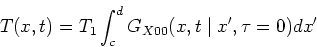(3)

Using the X00 Green's function evaluated at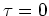,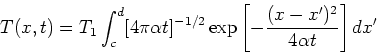(4)

Using the substitution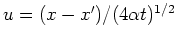this integral can be written as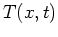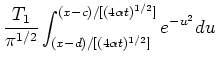(5)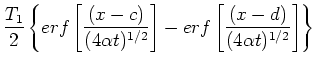(6)

where the error function is defined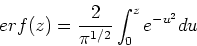(7)Next: Semi-infinite body heated at Up: EXAMPLES, TEMPERATURE FROM GF Previous: EXAMPLES, TEMPERATURE FROM GF
2004-01-31